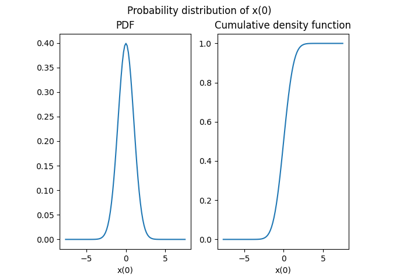gemseo / uncertainty / distributions / openturns

# normal module¶

Class to create a normal distribution from the OpenTURNS library.

This class inherits from OTDistribution.

class gemseo.uncertainty.distributions.openturns.normal.OTNormalDistribution(variable, mu=0.0, sigma=1.0, dimension=1, transformation=None, lower_bound=None, upper_bound=None, threshold=0.5)[source]

Bases: OTDistribution

Create a normal distribution.

Example

>>> from gemseo.uncertainty.distributions.openturns.normal import (
...     OTNormalDistribution
>>> )
>>> distribution = OTNormalDistribution('x', -1, 2)
>>> print(distribution)
Normal(mu=-1, sigma=2)

Parameters:
• variable (str) – The name of the normal random variable.

• mu (float) –

The mean of the normal random variable.

By default it is set to 0.0.

• sigma (float) –

The standard deviation of the normal random variable.

By default it is set to 1.0.

• dimension (int) –

The dimension of the normal random variable.

By default it is set to 1.

• transformation (str | None) – A transformation applied to the random variable, e.g. ‘sin(x)’. If None, no transformation.

• lower_bound (float | None) – A lower bound to truncate the distribution. If None, no lower truncation.

• upper_bound (float | None) – An upper bound to truncate the distribution. If None, no upper truncation.

• threshold (float) –

A threshold in [0,1].

By default it is set to 0.5.

compute_cdf(vector)

Evaluate the cumulative density function (CDF).

Evaluate the CDF of the components of the random variable for a given realization of this random variable.

Parameters:

vector (Iterable[float]) – A realization of the random variable.

Returns:

The CDF values of the components of the random variable.

Return type:

ndarray

compute_inverse_cdf(vector)

Evaluate the inverse of the cumulative density function (ICDF).

Parameters:

vector (Iterable[float]) – A vector of values comprised between 0 and 1 whose length is equal to the dimension of the random variable.

Returns:

The ICDF values of the components of the random variable.

Return type:

ndarray

compute_samples(n_samples=1)

Sample the random variable.

Parameters:

n_samples (int) –

The number of samples.

By default it is set to 1.

Returns:

The samples of the random variable,

The number of columns is equal to the dimension of the variable and the number of lines is equal to the number of samples.

Return type:

ndarray

plot(index=0, show=True, save=False, file_path=None, directory_path=None, file_name=None, file_extension=None)

Plot both probability and cumulative density functions for a given component.

Parameters:
• index (int) –

The index of a component of the random variable.

By default it is set to 0.

• save (bool) –

If True, save the figure.

By default it is set to False.

• show (bool) –

If True, display the figure.

By default it is set to True.

• file_path (str | Path | None) – The path of the file to save the figures. If the extension is missing, use file_extension. If None, create a file path from directory_path, file_name and file_extension.

• directory_path (str | Path | None) – The path of the directory to save the figures. If None, use the current working directory.

• file_name (str | None) – The name of the file to save the figures. If None, use a default one generated by the post-processing.

• file_extension (str | None) – A file extension, e.g. ‘png’, ‘pdf’, ‘svg’, … If None, use a default file extension.

Returns:

The figure.

Return type:

Figure

plot_all(show=True, save=False, file_path=None, directory_path=None, file_name=None, file_extension=None)

Plot both probability and cumulative density functions for all components.

Parameters:
• save (bool) –

If True, save the figure.

By default it is set to False.

• show (bool) –

If True, display the figure.

By default it is set to True.

• file_path (str | Path | None) – The path of the file to save the figures. If the extension is missing, use file_extension. If None, create a file path from directory_path, file_name and file_extension.

• directory_path (str | Path | None) – The path of the directory to save the figures. If None, use the current working directory.

• file_name (str | None) – The name of the file to save the figures. If None, use a default one generated by the post-processing.

• file_extension (str | None) – A file extension, e.g. ‘png’, ‘pdf’, ‘svg’, … If None, use a default file extension.

Returns:

The figures.

Return type:

list[Figure]

dimension: int

The number of dimensions of the random variable.

distribution: type

The probability distribution of the random variable.

distribution_name: str

The name of the probability distribution.

marginals: list[type]

The marginal distributions of the components of the random variable.

math_lower_bound: ndarray

The mathematical lower bound of the random variable.

math_upper_bound: ndarray

The mathematical upper bound of the random variable.

property mean: ndarray

The analytical mean of the random variable.

num_lower_bound: ndarray

The numerical lower bound of the random variable.

num_upper_bound: ndarray

The numerical upper bound of the random variable.

parameters: tuple[Any] | dict[str, Any]

The parameters of the probability distribution.

property range: list[numpy.ndarray]

The numerical range.

The numerical range is the interval defined by the lower and upper bounds numerically reachable by the random variable.

Here, the numerical range of the random variable is defined by one array for each component of the random variable, whose first element is the lower bound of this component while the second one is its upper bound.

property standard_deviation: ndarray

The analytical standard deviation of the random variable.

standard_parameters: dict[str, str] | None

The standard representation of the parameters of the distribution, used for its string representation.

property support: list[numpy.ndarray]

The mathematical support.

The mathematical support is the interval defined by the theoretical lower and upper bounds of the random variable.

Here, the mathematical range of the random variable is defined by one array for each component of the random variable, whose first element is the lower bound of this component while the second one is its upper bound.

transformation: str

The transformation applied to the random variable, e.g. ‘sin(x)’.

variable_name: str

The name of the random variable.

## Examples using OTNormalDistribution¶Probability distributions based on OpenTURNS

Probability distributions based on OpenTURNS